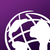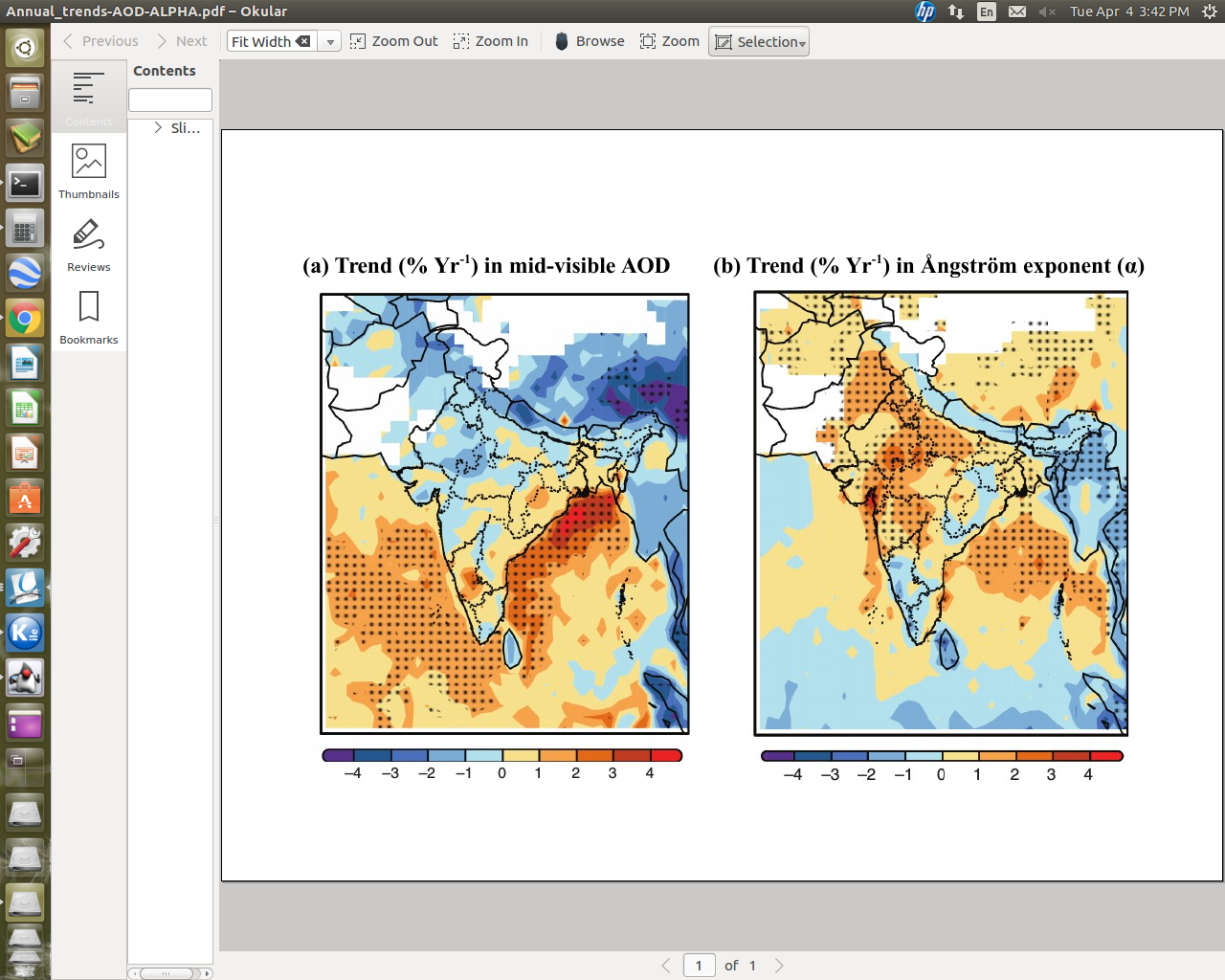# Plot Correlation coefficient along with Significant Value in Arc GIS 10.3

407
0
04-04-2017 03:37 AMOccasional Contributor III

Hi All,

I am working on two raster datasets, one raster is co-relation coefficient and another one is the significance of correlation.

I want to plot significance of correlation raster as a point, where the significance value more than > 90 % then it will create point feature on base map.

Meanwhile, here base map will be co-relation coefficient raster and significant of correlation raster will overlay as a pattern feature where > 90 significance value, then it will make point feature on the correlation raster. Below i am showing the images what i am trying to do,Above image shows correlation coefficient along with significant value  (By balck doted), where significant value > 90 , then point will create automatically else no point will create.

Thank you

Tags (4)
0 Replies